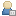###Show Posts

This section allows you to view all posts made by this member. Note that you can only see posts made in areas you currently have access to.

### Messages - shuxiang

Pages: 1 2 
51
##### RNA structures (DSSR) / Web DSSR for dissecting the spatial structures of nucleic acids
« on: July 29, 2018, 02:16:30 pm »
Web DSSR is a user-friendly web-based interface for analyzing and visualizing three-dimensional (3D) nucleic-acid-containing structures. The server allows the user to determine a wide variety of conformational parameters in a given PDB ID or uploaded structure. Meanwhile, the secondary structure visualization component offers simultaneously highlighting of 1D, 2D, and 3D nucleic-acid structures.

The wDSSR web server is located at http://web.x3dna-dssr.org/.

Any questions and bug reports are welcome!

52
##### RNA structures (DSSR) / Re: DSSR-enhanced visualization web server cannot display ribosome structures?
« on: May 17, 2018, 12:58:25 pm »
Thank you for the explanation.

53
##### RNA structures (DSSR) / Re: DSSR-enhanced visualization web server cannot display ribosome structures?
« on: May 17, 2018, 12:08:56 pm »
Thank you so much for your quick response. Just now I did a test and found a interesting thing.
I uploaded the 5afi.cif file (26MB) to the web server from a local machine, it works.
I fetched 5afi ID directly in the web server, it failed.

54
##### RNA structures (DSSR) / DSSR-enhanced visualization web server cannot display ribosome structures?
« on: May 17, 2018, 11:31:00 am »
Dear Xiangjun,

I used DSSR-enhanced visualization web server to display ribosome structures such as 5afi, 5j4b. All my tried failed. I'm not sure it is my browser problem or the web server inner error. Thank you.

Best,
Shuxiang

55
##### RNA structures (DSSR) / Re: How to display RNA secondary structure with & in forna?
« on: May 15, 2018, 11:35:00 am »
It works. Thank you so much.56
##### RNA structures (DSSR) / How to display RNA secondary structure with & in forna?
« on: May 15, 2018, 11:10:38 am »
Dear Xiangjun,

I tried to use the following dbn file generated by DSSR and display its RNA secondary structure in forna (a RNA secondary structure visualization tool). However, it looks like forna doesn't recognize multiple sequences and "&" notation. It there a way to get around this? Thank you.

>2F4V nts=1511 [2F4V] -- secondary structure derived by DSSR
UGGAGAGUUUGAUCCUGGCUCAGGGUGAACGCUGGCGGCGUGCCUAAGACAUGCAAGUCGUGCGGG&CCGCGGGGUUUU&ACUCCG&UGGUC&AGCGGCGGACGGGUGAGUAACGCGUGGGUGACCUACCCGGAAGAGGGGGACAACCCGGGGAAACUCGGGCUAAUCCCCCAUGUGGACCCGCCCCUUGGGGUGUGUCCAAAGGGCUUU&GCCCGCUUCCGGAUGGGCCCGCGUCCCAUCAGCUAGUUGGUGGGGUAAUGGCCCACCAAGGCGACGACGGGUAGCCGGUCUGAGAGGAUGGCCGGCCACAGGGGCACUGAGACACGGGCCCCACUCCUACGGGAGGCAGCAGUUAGGAAUCUUCCGCAAUGGGCGCAAGCCUGACGGAGCGACGCCGCUUGGAGGAAGAAGCCCUUCGGGGUGUAAACUCCUGAA&CCCGGGACGAAACCCCCGACGA&GGGGACUGACGGUACCGGG&GUAAUAGCGCCGGCCAACUCCGUGCCAGCAGCCGCGGUAAUACGGAGGGCGCGAGCGUUACCCGGAUUCACUGGGCGUAAAGGGCGUGUAGGCGGCCUGGGGCGUCCCAUGUGAAAGACCACGGCUCAACCGUGGGGGAGCGUGGGAUACGCUCAGGCUAGACGGUGGGAGAGGGUGGUGGAAUUCCCGGAGUAGCGGUGAAAUGCGCAGAUACCGGGAGGAACGCCGAUGGCGAAGGCAGCCACCUGGUCCACCCGUGACGCUGAGGCGCGAAAGCGUGGGGAGCAAACCGGAUUAGAUACCCGGGUAGUCCACGCCCUAAACGAUGCGCGCUAGGUCUCUGGGUCU&CCUGGGGGCCGAAGCUAACGCGUUAAGCGCGCCGCCUGGGGAGUACGGCCGCAAGGCUGAAACUCAAAGGAAUUGACGGGGGCCCGCACAAGCGGUGGAGCAUGUGGUUUAAUUCGAAGCAACGCGAAGAACCUUACCAGGCCUUGACAUGCUAGGGAACCCGGGUGAAAGCCUGGGGUGCCCCGCGAGGGGAGCCCUAGCACAGGUGCUGCAUGGCCGUCGUCAGCUCGUGCCGUGAGGUGUUGGGUUAAGUCCCGCAACGAGCGCAACCCCCGCCGUUAGUUGCCAGCGGUUCGGCCGGGCACUCUAACGGGACUGCCCGCGAAAGCGGGAGGAAGGAGGGGACGACGUCUGGUCAGCAUGGCCCUUACGGCCUGGGCGACACACGUGCUACAAUGCCCACUACAAAGCGAUGCCACCCGGCAACGGGGAGCUAAUCGCAAAAAGGUGGGCCCAGUUCGGAUUGGGGUCUGCAACCCGACCCCAUGAAGCCGGAAUCGCUAGUAAUCGCGGAUCAGCCAUGCCGCGGUGAAUACGUUCCCGGGCCUUGUACACACCGCCCGUCACGCCAUGGGAGCGGGCUCUACCCGAAGUCGCCGGG&AGCCUACGGG&CAGGCGCCGAGGGUAGGGCCCGUGACUGGGGCGAAGUCGUAACAAGGUAGCUGUACCGGAAGGUGCGGCUGGAUC&A&UUCU
....((((..[.[[[..)))).((((.(((((..(((((((((....(((.(((..(((..((.((&((((((((....&.)))))&)))))&.)))))......(((......((((((((..((...(((((((.(((((....((((((....)))))).....)))))....((((.(((((....))))).))))...((((...&)))).)))))))..))))))))))(((....(((..((((((((.......)))))))))))......)))..((((((((....))))...))))))).(((((............))))).((((....))))...)))))).).....(.(((...((.((....)).).))))).)).))))))..((((.......(((....)))......))))....&(.(((...(....((((.....&)))).....)....))).)&......((((([[[...(((((.....((.]]])).......))))))))))..)))))))))..........((([[...(.((((...(((.(((((((.((((((((((......((((((.....))))))....))))))))..)))))))))..(((.(((((...((((((((...(((((((....((........)).......)))))))...).......((....)).)))))))..)))))..))..))))...))))....((((((...((...((((.........))))...))))))))......{...((((((..((((((((((...&))))))))))...((..]])).....)))))))))).(((......((((....))))....)))...]]].](((((.(((((((.((..(((((..((((((((((......((........))..........((((((...(...((............(.(....).)........(((....).))........))).((.(((...((((((.(....(((((((((....)))..((((......))))..)))))).....((((.(((((.....(....(.......)..)......)))))..(..(((((....))))).....)..)))).....).).)))...)).))))).....))))))..[)).)))))))).(...(((((((.....(((..((..((((....))))..))....))).....)))))))......(....(((((((........)))))))....)..)..))))).....(((((((......]...)))))))......))...)))))))))).))..(.(..((.(.((((.(((..((.(((.((((((...(.((((...&.(((....))&).)))).)..)))))).))).))..))).))))..).))...)..)..(((((((((....)))))))))}....&.&....

Best,
Shuxiang

Pages: 1 2 

Created and maintained by Dr. Xiang-Jun Lu [律祥俊], Principal Investigator of the NIH grant R01GM096889
Dr. Lu is currently affiliated with the Bussemaker Laboratory at the Department of Biological Sciences, Columbia University.